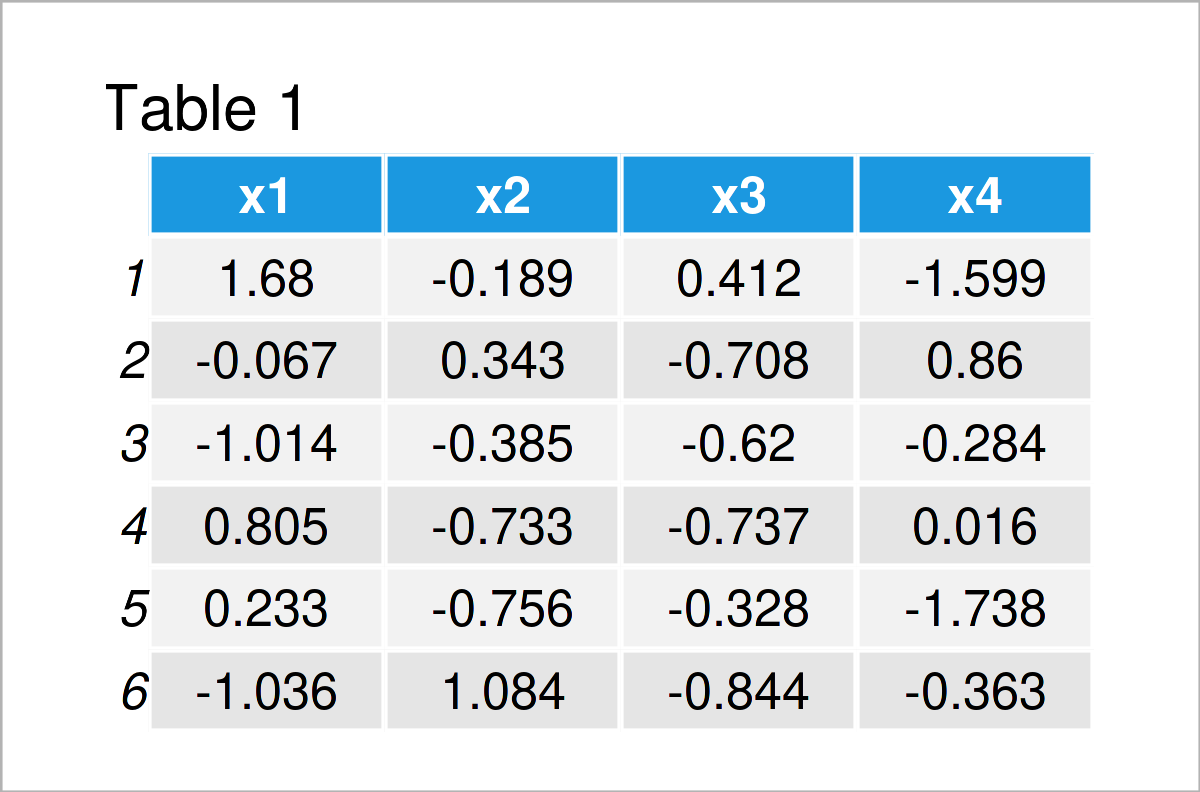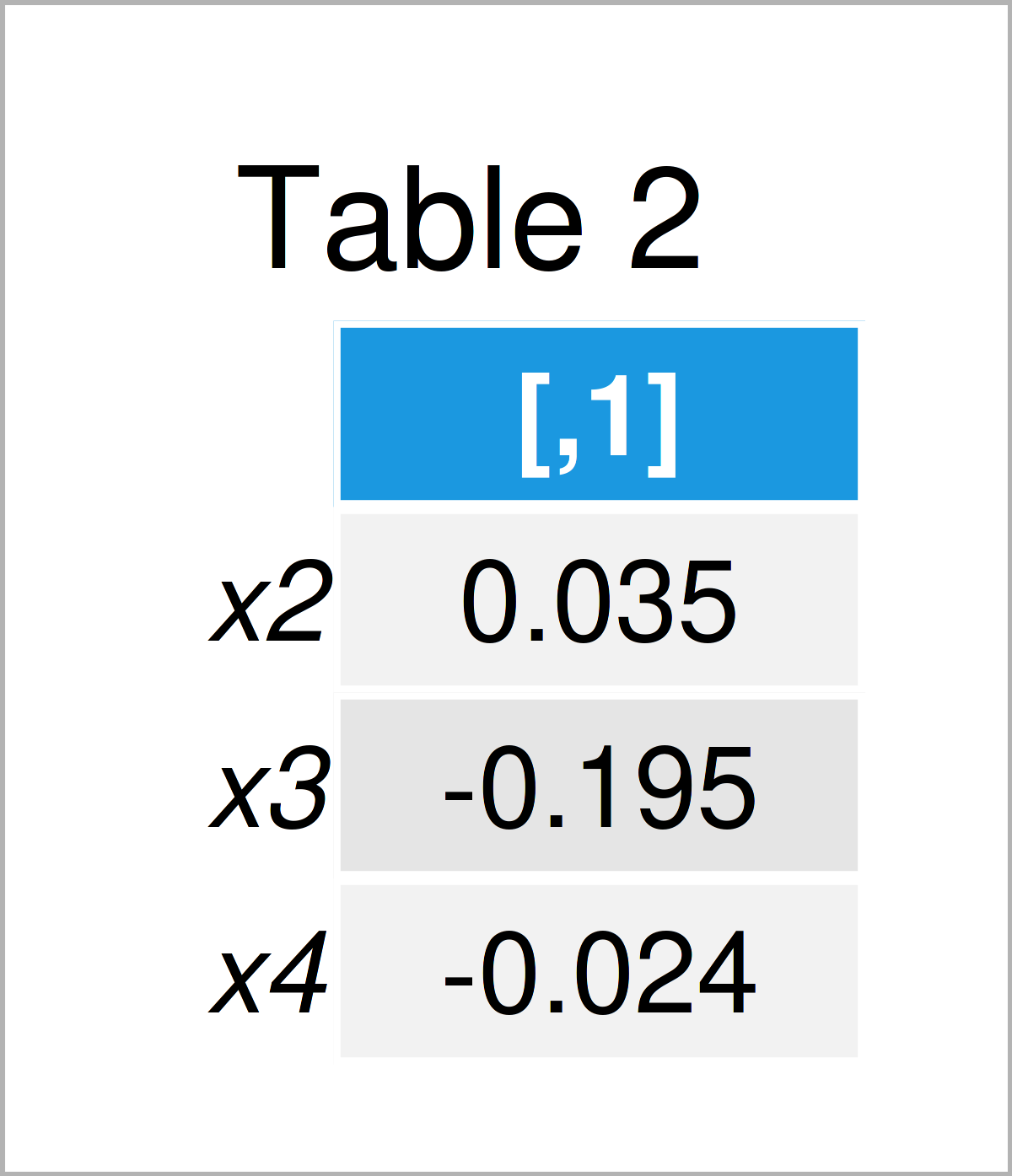# Correlation of One Variable to All Others in R (Example)

In this R tutorial you’ll learn how to calculate the correlation of one data frame column to all the others.

The page contains this:

It’s time to dive into the example…

## Creation of Exemplifying Data

Consider the following example data:

```set.seed(6529489)                                 # Create example data
data <- data.frame(x1 = rnorm(100),
x2 = rnorm(100),
x3 = rnorm(100),
x4 = rnorm(100))Table 1 shows that our example data is constructed of the four columns “x1”, “x2”, “x3”, and “x4”.

## Example: Calculate Correlation of One Variable to All Others Using cor() Function

In this example, I’ll demonstrate how to get the Pearson correlation coefficient between a particular data frame variable with all the other variables in this data frame.

To achieve this, we can apply the cor and colnames functions as shown below:

```data_cor <- cor(data[ , colnames(data) != "x1"],  # Calculate correlations
data\$x1)
data_cor                                          # Print correlation values```As visualized in Table 2, the previous code has managed to construct a correlation matrix for only one of the columns in our data set.

## Video, Further Resources & Summary

If you need more information on the R codes of this article, you might watch the following video on my YouTube channel. I demonstrate the R programming codes of this tutorial in the video:

Please accept YouTube cookies to play this video. By accepting you will be accessing content from YouTube, a service provided by an external third party.If you accept this notice, your choice will be saved and the page will refresh.

In addition, you may read the related articles on Statistics Globe. A selection of articles about topics such as ggplot2, variables, lists, and graphics in R is shown below.

This tutorial has demonstrated how to compute the correlation of one data frame column to many others in R. If you have additional questions and/or comments, please let me know in the comments below.

Subscribe to the Statistics Globe Newsletter

•Vanessa
April 12, 2023 1:35 pm

Hey thank you for the code, it works fine. I need the significances, too, but cor.mtest doesnt work.Do you have any idea, how to calculate the coefficients and sgnificances?

•April 13, 2023 8:01 am

Hello Vanessa,

You need to convert your data frame to a matrix to use the cor.mtest() function. First, be sure that you install the library:

```install.packages("corrplot")
library(corrplot)```

Then convert your data to a matrix:

`data_matrix <- as.matrix(data)`

After that, you can run the function:

`cor.mtest(data_matrix)`

Regards,
Cansu

•Katharina
August 28, 2023 11:50 am

How can I then plot a corrplot including significant marks (or only circles when significant) for the correlation of x1 with all the other variables (x2, x3 and x4)? resulting in a correlation “vector”

•August 28, 2023 12:56 pm

Hello Katharina,

Would a plot like here in the second example help? See the title Use chart.Correlation(): Draw scatter plots.

Best,
Cansu

•Zach
September 7, 2023 2:29 pm

Is there a way to make this work with cor.test as well? Thanks!

•September 8, 2023 12:09 pm

Hello Zach,

I understand that you are intention is to test the correlation between a variable and the others. You can achieve this by using a defined function in the apply() procedure. See the following script.

```# Create a sample dataframe
df &lt;- data.frame(var1 = rnorm(100),
var2 = rnorm(100),
var3 = rnorm(100),
var4 = rnorm(100))

# Test correlation of var1 with all other variables
results &lt;- lapply(names(df)[-1], function(col_name) {
test_result &lt;- cor.test(df\$var1, df[[col_name]])
list(column = col_name,
cor_coefficient = test_result\$estimate,
p_value = test_result\$p.value)
})

# Print the results
results```

Best,
Cansu